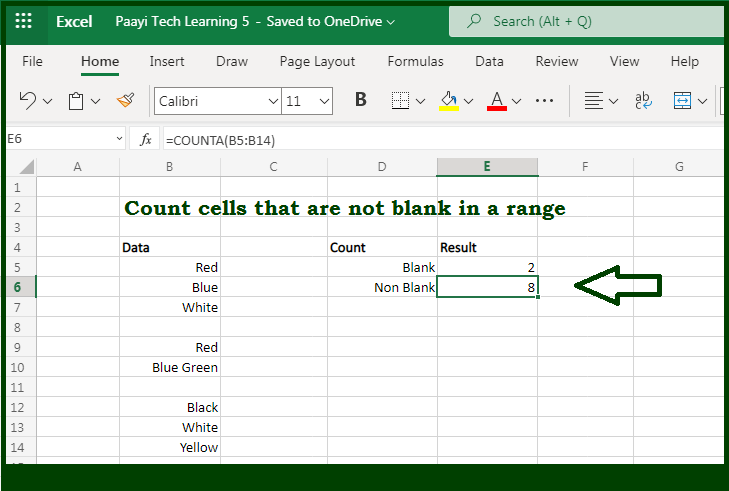# Learn How to Count Cells that are Not Blank in Microsoft Excel

Written by | 0 Comments | 480 Views

In this article, you will learn how to COUNT various things in Microsoft Excel using a single or combination of functions and its purpose in Microsoft Excel. You will also get to know how to count cells that are not blank and see the generic formula.

Count Cells that are Not Blank in Microsoft Excel

The main purpose of this formula is to count cells that are not blank. Here we will learn how to count cells that contain some data in Microsoft Excel.  That implies, with the help of a formula based on the  COUNTA function you can able to count cells that contain some kind of data. So, with the help of this formula, you can able to count cells that are not blank.

General Formula to COUNT Cells that are Not Blank

=COUNTA(range)

The Explanation for the COUNT Cells that are Not BlankSo we know that with the help of the given formula above you can able to count cells that are not blank. Here we will learn how to count cells that contain some data in Microsoft Excel. As we know that, the COUNTA function is fully automatic. When the range of cells is provided, it returns a count of cells that contain numbers, text, logical values, and errors. It should be noted that empty cells are ignored. So, with the help of this formula, you can able to count cells that are not blank.